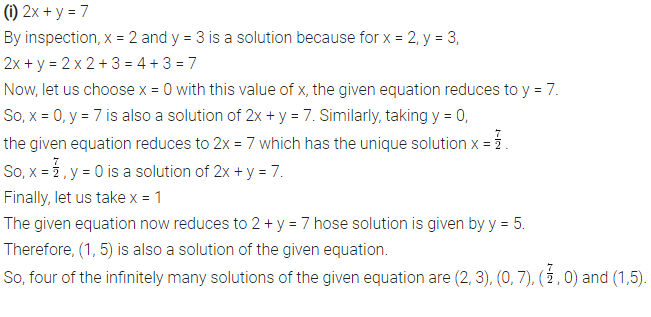# Write four solutions for each of the following equations

Write four solutions for each of the following equations
(i) 2x + y = 7
(ii) πx + y = 9
(iii) x = 4y